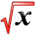# Areas and DistancesThis article is part of the MathHelp Tutoring Wiki

## Areas and Distances

1. AREA

-Calculating area under the curve using Reimann Sum: dividing the area into rectangular strips. The more rectangular strips, the closer the approximation will be to the area.

-Width of the rectangles: Δx=b-a/n

Sigma notation (Σ) is used for summation of many terms

Sigma Notation: (c=constant)

Σc=nc

Σca= cΣa

Σ(a+b)=Σa+Σb

Σ(a-b)=Σa-Σb

### Some formulas

Σ i= n(n+1)/2

Σ i2= n(n+1) (2n+1)/6

Σ i3=(n(n+1)/2)2

Example 1:

```   * Back to Integral Calculus
* Back to MathHelp
```